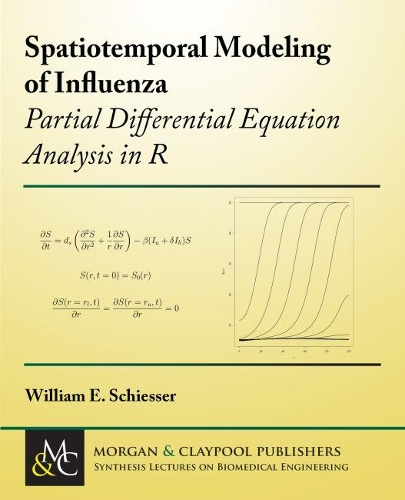•# Spatiotemporal Modeling of Influenza: Partial Differential Equation Analysis in R - Synthesis Lectures on Biomedical Engineering (Paperback)

(author)
£49.50
Paperback 111 Pages / Published: 30/04/2019
• We can order this

Usually dispatched within 3 weeks

This book has a two-fold purpose:An introduction to the computer-based modeling of influenza, a continuing major worldwide communicable disease.The use of (1) as an illustration of a methodology for the computer-based modeling of communicable diseases.

For the purposes of (1) and (2), a basic influenza model is formulated as a system of partial differential equations (PDEs) that define the spatiotemporal evolution of four populations: susceptibles, untreated and treated infecteds, and recovereds. The requirements of a well-posed PDE model are considered, including the initial and boundary conditions. The terms of the PDEs are explained.

The computer implementation of the model is illustrated with a detailed line-by-line explanation of a system of routines in R (a quality, open-source scientific computing system that is readily available from the Internet). The R routines demonstrate the straightforward numerical solution of a system of nonlinear PDEs by the method of lines (MOL), an established general algorithm for PDEs.

The presentation of the PDE modeling methodology is introductory with a minumum of formal mathematics (no theorems and proofs), and with emphasis on example applications. The intent of the book is to assist in the initial understanding and use of PDE mathematical modeling of communicable diseases, and the explanation and interpretation of the computed model solutions, as illustrated with the influenza model.

Publisher: Morgan & Claypool Publishers
ISBN: 9781681735696
Number of pages: 111
Dimensions: 235 x 191 mm